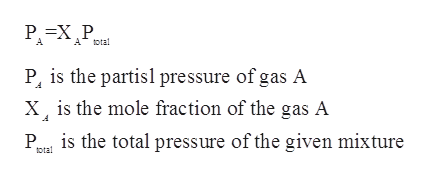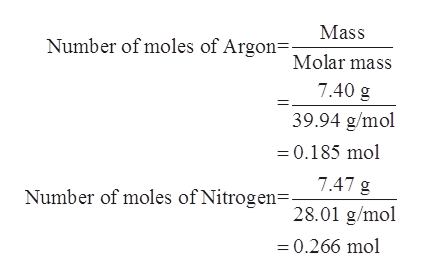# A mixture of argon and nitrogen gases, at a total pressure of 965 mm Hg, contains 7.40 grams of argon and 7.47 grams of nitrogen. What is the partial pressure of each gas in the mixture?PAr =  __mm HgPN2 = __ mm Hg

Question
7 views

A mixture of argon and nitrogen gases, at a total pressure of 965 mm Hg, contains 7.40 grams of argon and 7.47 grams of nitrogen. What is the partial pressure of each gas in the mixture?

PAr =  __mm Hg
PN2 = __ mm Hg

check_circle

Step 1

According to the Dalton’s law of partial pressures, in a given mixture containing non-reacting gases, the total pressure exerted is equal to the sum of the partial pressures which is exerted by the individual gases in the mixture.

Step 2

The partial pressure of a given as is equal to the product of the mole fraction of the gas and the total pressure exerted by the gases present in the mixture.help_outlineImage TranscriptioncloseA otal P, is the partisl pressure of gas A X is the mole fraction of the gas A Pris the total pressure of the given mixture tota fullscreen
Step 3

The mass of Argon gas is given to be 7.40 g.

The mass of Nitrogen gas is given to be 7.47 g...help_outlineImage TranscriptioncloseMass Number of moles of Argon= Molar mass 7.40 g 39.94 g/mol 0.185 mol 7.47 g Number of moles of Nitrogen=- 28.01 g/mol 0.266 mol fullscreen

### Want to see the full answer?

See Solution

#### Want to see this answer and more?

Solutions are written by subject experts who are available 24/7. Questions are typically answered within 1 hour.*

See Solution
*Response times may vary by subject and question.
Tagged in

### Physical Chemistry